﻿

### TheNeumann Laplacian on spaces of continuous functions

#### Abstract

If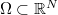$\Omega \subset \mathbb{R}^N$ is an open set, one can always define the Laplacian with Neumann boundary conditions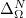$\Delta^N_\Omega$ on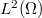$L^2(\Omega)$. It is a self-adjoint operator generating a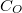$C_O$-semigroup on$L^2(\Omega)$. Considering the part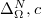$\Delta^N_\Omega,c$ of$\Delta^N_\Omega$ in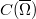$C(\overline{\Omega})$,we ask under which conditions on it generates a$C_O$-semigroup.

DOI Code: 10.1285/i15900932v22n1p65

Keywords: Neumann Laplace

Full Text: PDF